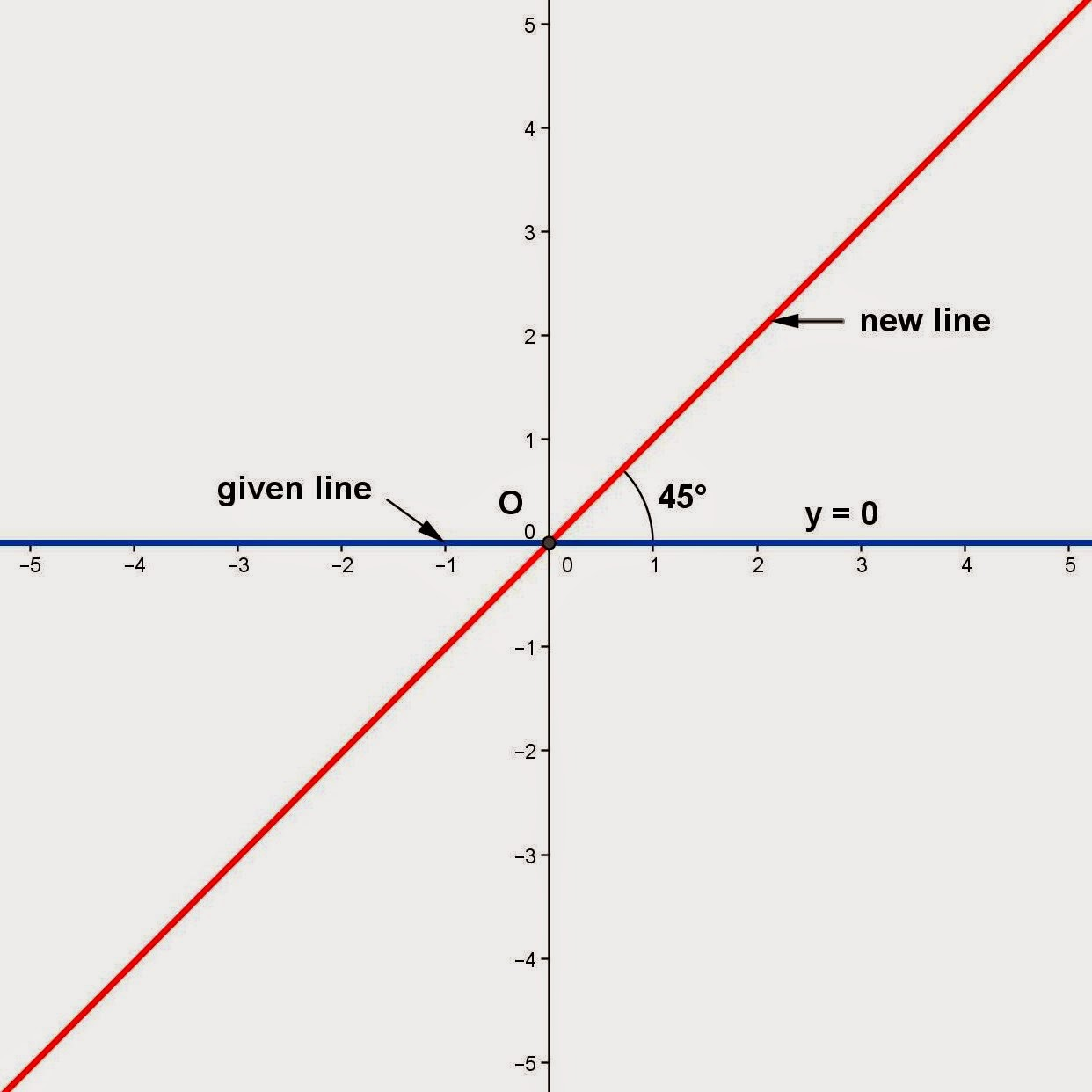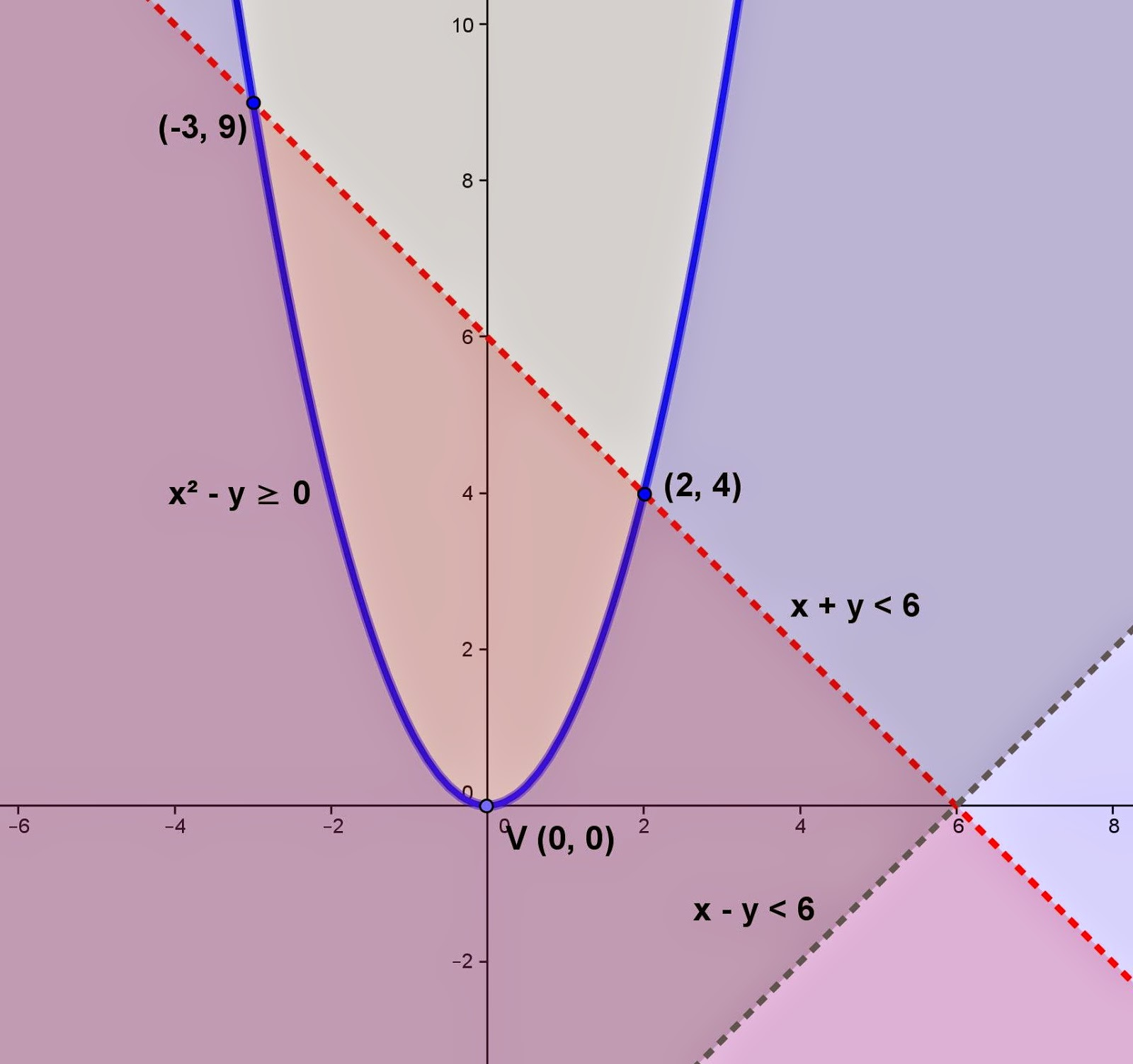## Friday, October 31, 2014

### Rotation of a Line

Category: Analytic Geometry

"Published in Vacaville, California, USA"

Given the equation of a line

Find the new equation of a line if the given line is rotated about the origin at 45°.

Solution:

To illustrate the given problem, it is better to draw the figure as followsPhoto by Math Principles in Everyday Life

If the given equation is written in rectangular coordinate system, then we need to convert it into polar coordinate system as follows

Next, substitute θ with θ - 45° and then expand using the sum and difference of two angles formula, we have

Convert the above equation into rectangular coordinate system in order to get its final equation. Therefore, the new equation of a line is

## Thursday, October 30, 2014

Category: Algebra

"Published in Newark, California, USA"

Find the roots of the equation for

Solution:

The first thing that we need to do is to examine the given equation first if we can simplify or not. In this case, 3y + 4 is a common factor on both sides of the equation. Cancel their common factor, we have

Since y² will be eliminated from the above equation, then it becomes a linear equation and we can solve for the value of y. The value of y is

Next, we need to check if the value of y is a root or not since the given equation is a radical equation. There are cases that the roots are extraneous. Let's check the value of y from the original equation, we have

Since both sides of the equation are equal, therefore, y = 6 is the root of the equation.

## Wednesday, October 29, 2014

### Finding the Equation of Ellipse, 2

Category: Analytic Geometry

"Published in Vacaville, California, USA"

Find the equation of ellipse with center C(0, 4), foci F1(0, 0) and F2(0, 8), and major axis of length 10 units.

Solution:

To illustrate the problem, it is better to draw the figure as followsPhoto by Math Principles in Everyday Life

If the coordinates of the center and foci and the length of major axis are given, then c = 4, and a = 10/2 = 5. The value of b is

Since the coordinates of the foci have the same x-coordinate, then the major axis is parallel to y-axis. Therefore, the equation of ellipse whose center is C(h, k) in standard form is

In general form, the equation of ellipse is

## Tuesday, October 28, 2014

### Word Problem - System of Inequalities

Category: Analytic Geometry

"Published in Vacaville, California, USA"

A publishing company publishes a total of no more than 100 books every year. At least 20 of these are non-fiction, but the company always publishes at least as much fiction as non-fiction. Find a system of inequalities that describes the possible numbers of fiction and non-fiction books that the company can produce each year consistent with these policies. Graph the solution set.

Solution:

The given word problem is about getting the number of fiction and non-fiction books by sketching the graph of the system of inequalities.

Let x = be the number of fiction books
y = be the number of non-fiction books

From the word statement "A publishing company publishes a total of no more than 100 books every year.", then the working equation will be

Since the sign of inequality is less than or equal to, then all points along the line are included in the solution. If x = 0 and y = 0, then the given equation reduces to 0 ≤ 100 which is correct and that point is included in the solution.

From the word statement "At least 20 of these are non-fiction,..", then the working equation will be

Since the sign of inequality is greater than or equal to, then all points along the line are included in the solution. However, all points above the given line are included in the solution.

From the word statement "...but the company always publishes at least as much fiction as non-fiction.", then the working equation will be

Since the sign of inequality is greater than or equal to, then all points along the line are included in the solution. If x = 20 and y = 10, then the given equation reduces to 20 ≥ 10 which is correct and that point is included in the solution. Therefore, the graph of a set of inequalities isPhoto by Math Principles in Everyday Life

The number of fiction and non-fiction books which are the coordinates of the vertices are (20, 20), (50, 50), and (80, 20). The three vertices are located at the intersection of the three shaded regions bounded by three lines.

## Monday, October 27, 2014

### Graph of the System of Inequalities, 5

Category: Analytic Geometry

"Published in Vacaville, California, USA"

Graph the solution of the system of inequalities and find the coordinates of all vertices for

a. x² + y² ≤ 8
x ≥ 2
y ≥ 0

b. x² - y ≥ 0
x + y < 6
x - y < 6

Solution:

a. For x² + y² ≤ 8, the given equation is a circle whose center is C(0, 0) and r = 8 = 22. Since the sign of inequality is less than or equal to, then all points along the curve are included in the solution. If x = 0 and y = 0, then the given equation reduces to 0 ≤ 8 which is correct and that point is included in the solution.

For x ≥ 2, the given line is a vertical line in which all points along the line are included in the solution. However, all points at the right of the given line are included in the solution.

For y ≥ 0, the given line is a horizontal line in which all points along the line are included in the solution. However, all points above of the given line are included in the solution.  Therefore, the graph of a set of inequalities isPhoto by Math Principles in Everyday Life

The vertices of the graph are (2, 2), (2, 0) and (22, 0) that are located at the intersection of the three shaded regions bounded by two lines and a circle. They are also included in the solution.

b. For x² - y ≥ 0, the given equation is a parabola that concave upward whose vertex is V(0, 0). Since the sign of inequality is greater than or equal to, then all points along the curve are included in the solution. If x = 0 and y = 0, then the given equation reduces to 0 ≥ 0 which is correct and that point is included in the solution.

For x + y < 6, we need to rewrite the given equation into slope-intercept form as follows

Since the sign of inequality is less than, then all points along the line are not included in the solution. If x = 0 and y = 0, then the given equation reduces to 0 < 6 which is correct and that point is included in the solution.

For x - y < 6, we need to rewrite the given equation into slope-intercept form as follows

If you divide both sides of the equation by a negative number, then the sign of inequality will be reversed. Since the sign of inequality is less than, then all points along the line are not included in the solution. If x = 0 and y = 0, then the given equation reduces to 0 < 6 which is correct and that point is included in the solution. Therefore, the graph of a set of inequalities isPhoto by Math Principles in Everyday Life

The vertices of the graph are (-3, 9) and (2, 4) that are located at the intersection of the three shaded regions bounded by two lines and a parabola. They are also included in the solution.